# Geometric Series

A geometric series is the sum of some or all of the terms of a geometric sequence.

# Example 1

Consider the series: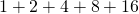Now, there are only 5 terms here, and so the answer is not difficult to compute. However, the principles demonstrated with this series can be used for any number of terms on any complexity of geometric sequence.

The following applet is rather unwieldy and might not fit into a smaller screen. Geometric sequences do become unwieldy quickly. The applet seeks to explain the mathematical process that is captured in the formula for summing a geometric sequence. The formula will be given at the end of this page.

# Example 2

Here is another example, this time summing the first seven terms of a geometric sequence:

A geometric sequence begins:The sum of the first seven terms is:.

Again, because there are only seven terms, it isn’t unreasonable to go ahead and add them together.

But let’s do it in a clever way!

First, lets give the sum a name. As we are adding seven terms, we will call is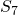.

Next, we will multiply each term by the common ratio. So we will have.

Then, we will subtractfrom, leaving us with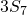and a very simple expression. Let’s go ahead and do that: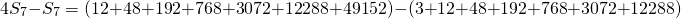Notice that on the left hand side, we just have, and on the right hand side all but two of the terms will cancel out.Simplifying to:This is a tidy calculation to preform: we find that.

# Generalizing

To generalize, let’s review the first seven terms of a geometric sequence:Notice that the first term is not multiplied by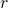and that the seventh term has.

Now, if we multiplyby the common ratiowe have: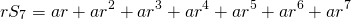Subtracting like we did before in the example we haveAgain, all but two terms on the right hand side cancel out.Let’s divide through by:Now factor out the common factoron the numerator:Finally, we are using 7 terms but we could be adding any positive integerterms. Let’s replace the 7 with:And there is the formula.

# Using the various formulae

Find eleven questions to practice this math at the end of this mathisfun page!

I’m done! Take me back to the Sequences and Series Menu!